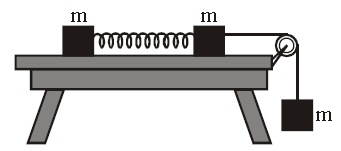# Calculating distances might not be easyOn a smooth horizontal table are two identical cubes of mass $m$, connected by a spring of spring constant $k$. The length of spring in the unstretched state is $L$. The right cube is linked to the load mass $m$ at the end. At some time the system is released and the system moves without initial velocity. Find the maximum distance (in $\text{cm}$) between blocks during the motion of the system.

[$L = 1 \text{ cm}, m = 3 \text{ kg}, k = 1000 \text{ N/m}$].

×